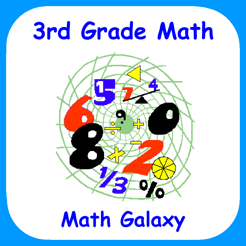## Screenshots

•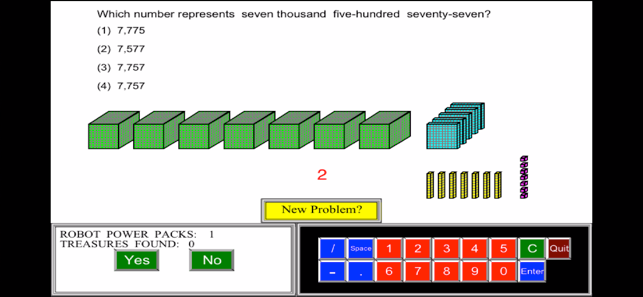•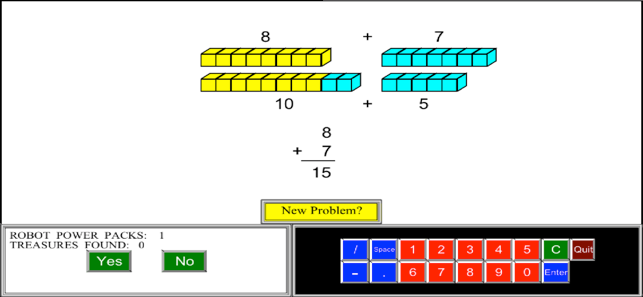•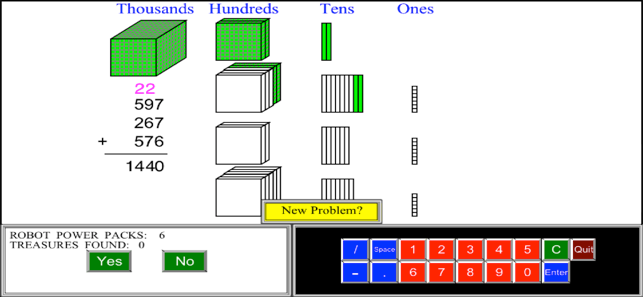•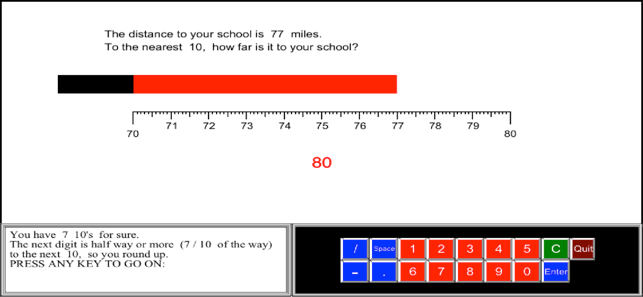•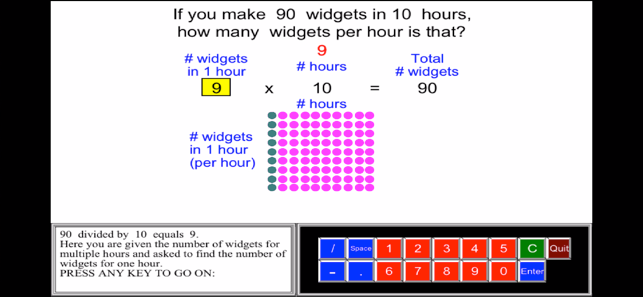•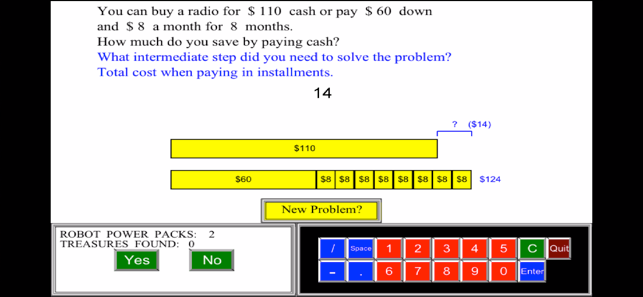•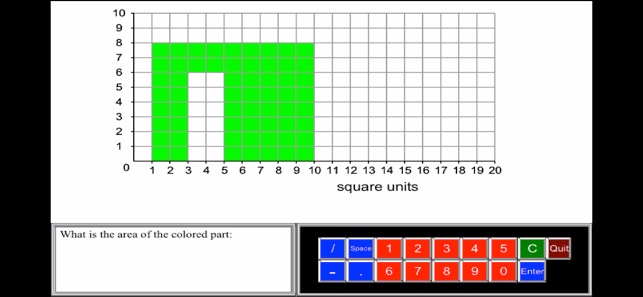•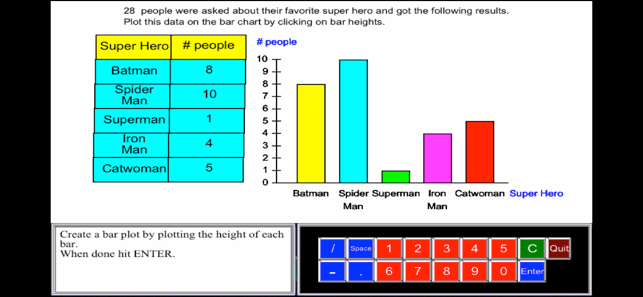•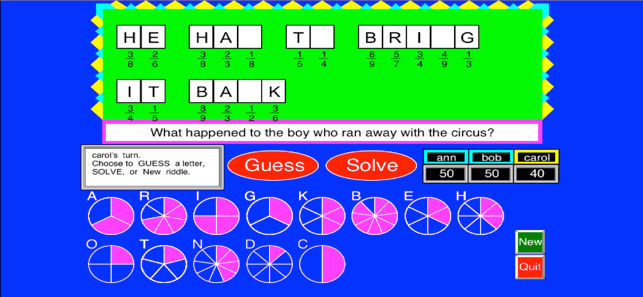•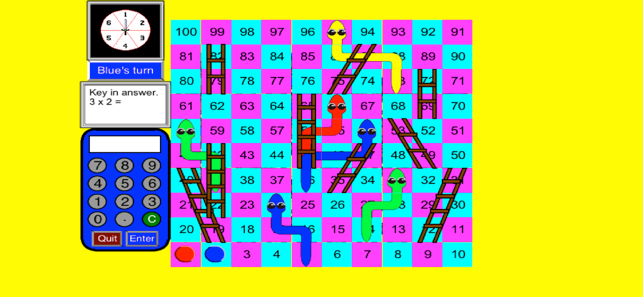## Description

"The French mathematician Jacques S. Hadamard found, in a study of 100 leading mathematicians [including Einstein], that the majority of them were primarily visual in their approach."
Scientific American, Sept., 1984, p. 56.

The secret to learning math is consistency.
Ten minutes or ten problems a day, if done consistently, can yield significant results.

Math Galaxy’s Third Grade Math covers all of the Common Core math standards for 3rd grade as listed below.
The concepts are presented in bite-size pieces and there is an unlimited supply of practice problems, with numbers chosen randomly.
Illustrations are used throughout to reinforce the concepts.
There are review sections and you can be guided through problems step-by-step or just give the final answer.
By solving problems students earn robots to use in a maze game, plus there are several other games involving math practice.
Certificates with scores and summaries of scores can be emailed to be printed.
A stylus is recommended for iPhones.

NUMBERS AND OPERATIONS IN BASE 10 (3.NBT)

Use place value understanding and properties of operations to perform multi-digit arithmetic.

Place Value (3.NBT.1)

Place Value Blocks
Place Names
Expanded Notation
Comparing Numbers
Ordering Numbers

Use place value understanding to round whole numbers to the nearest 10 or 100.

Rounding & Estimating

Rounding Whole Numbers ((3.NBT.1)
Estimating Whole Numbers (3.NBT.3)

Fluently add and subtract within 1000 using strategies and algorithms based on place value, properties of operations, and/or relationship between addition and subtraction.

Alternative Method - Partial Sums
Alternative Method - Expanded Notation

Subtracting Whole Numbers (3.NBT.2)

Subtraction on the Number Line
1-Digit Subtraction
2-Digit Subtraction
3-Digit Subtraction
Borrowing Across 0’s
Missing Number
Alternative Method

OPERATIONS AND ALGEBRAIC THINKING (3.OA)

Understand properties of multiplication and the relationship between multiplication and division.
Represent and solve problems involving multiplication and division.
Multiply and divide within 100.

Multiplication Tables (3.OA.7)
1-Digit Multiplication (3.OA.1)
Division Tables (3.OA.7)
Relating Division to Multiplication (3.OA.2, 6)
Relating Division Word Problems (3.OA.3, 4, 6)
1-Digit Divisor (3.OA.2)

Solve problems involving the four operations.

Word Problems (3.OA)

Multiply or Divide (3.OA.3)
Add, Subtract, Multiply, or Divide (3.OA.3, 6)
Multi-Step (3.OA.3, 8)

Identify and explain patterns in arithmetic.

Patterns (3.OA.9)
Skip Counting
Number & Shape Patterns

Algebraic Properties (3.OA.5)

NUMBER AND OPERATIONS - FRACTIONS (3.NF)

Develop understanding of fractions as numbers.

Identifying Fractions (3.NF.1)
Fractions on a Line (3.NF.2)
Drawing Fractions (3.NF.2)
Part of a Group (3.NF.1)
Equivalent Fractions (3.NF.3)
Creating Equivalent Fractions (3.NF.3.a)
Finding Equivalent Fractions (3.NF.3.b)
Comparing Fractions (3.NF.3.d)

MEASUREMENT AND DATA (3.MD)

Solve problems involving intervals of time, liquid volumes, and masses of objects.
Understand concepts of area and perimeter and relate them to multiplication and addition.

Time (3.MD.1)
Length (3.MD.4)
Area & Perimeter (3.MD.5, 6, 7, 8)
Liquid Volume (3.MD.2)

Represent and interpret data.

Pictographs (3.MD.3)
Bar Graphs (3.MD.3, 4)

GEOMETRY (3.G)

Reason with shapes and their attributes.
Terminology

GAMES

Labyrinth
Bridge the Swamp
The Money Trail

## What’s New

Version 3.2

Fixed drawing of blocks in 3-Digit Addition.
Some cosmetic changes.

## Ratings and Reviews

1.0 out of 5
1 Rating

1 Rating

AleksaMaze ,

### Like it

As I'm studying at the university and I really need to pass the math exam I've decided to get this app. So I would say it was worth to buy it cos I'm making some progress and this app helps to solve different types of problems and it's very useful if you try all of them week by week. This thing works.

malka987 ,

### not boring

You know this app is better than those boring exercise books. I spend my time with my beloved phone and do exercises. By the way they are not bad, I'd even say I like them.

Andrew_bazzz ,

This app helps my brother to do well at math lessons. He used to be very bad at maths but now he is very clever. His teacher is very proud of him.

## Information

Seller
Gilbert Reilly
Size
324.6 MB
Category
Education
Compatibility

Requires iOS 8.0 or later. Compatible with iPhone, iPad, and iPod touch.

Languages

English

Age Rating
4+
Price
\$4.99

## Supports

•